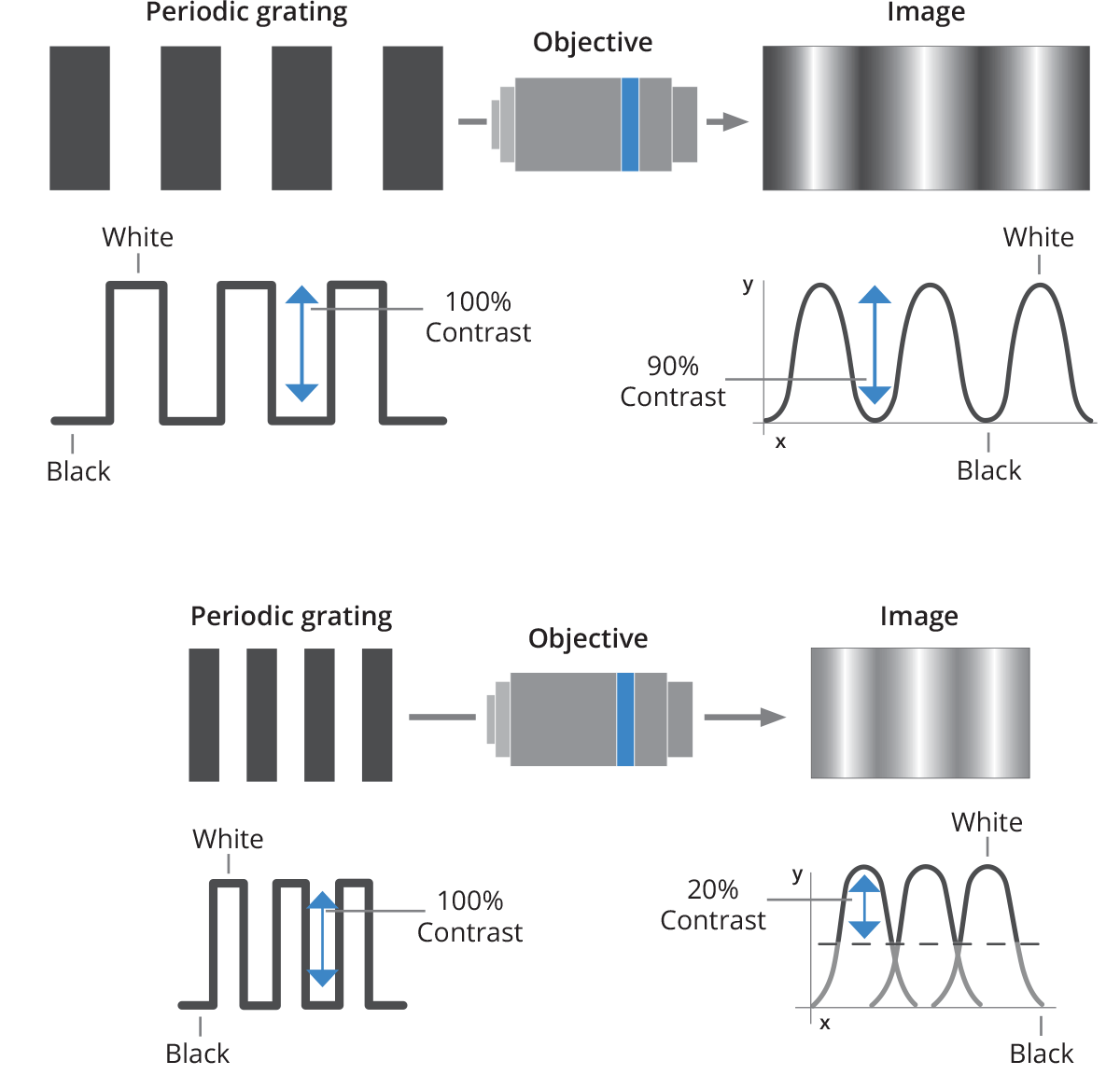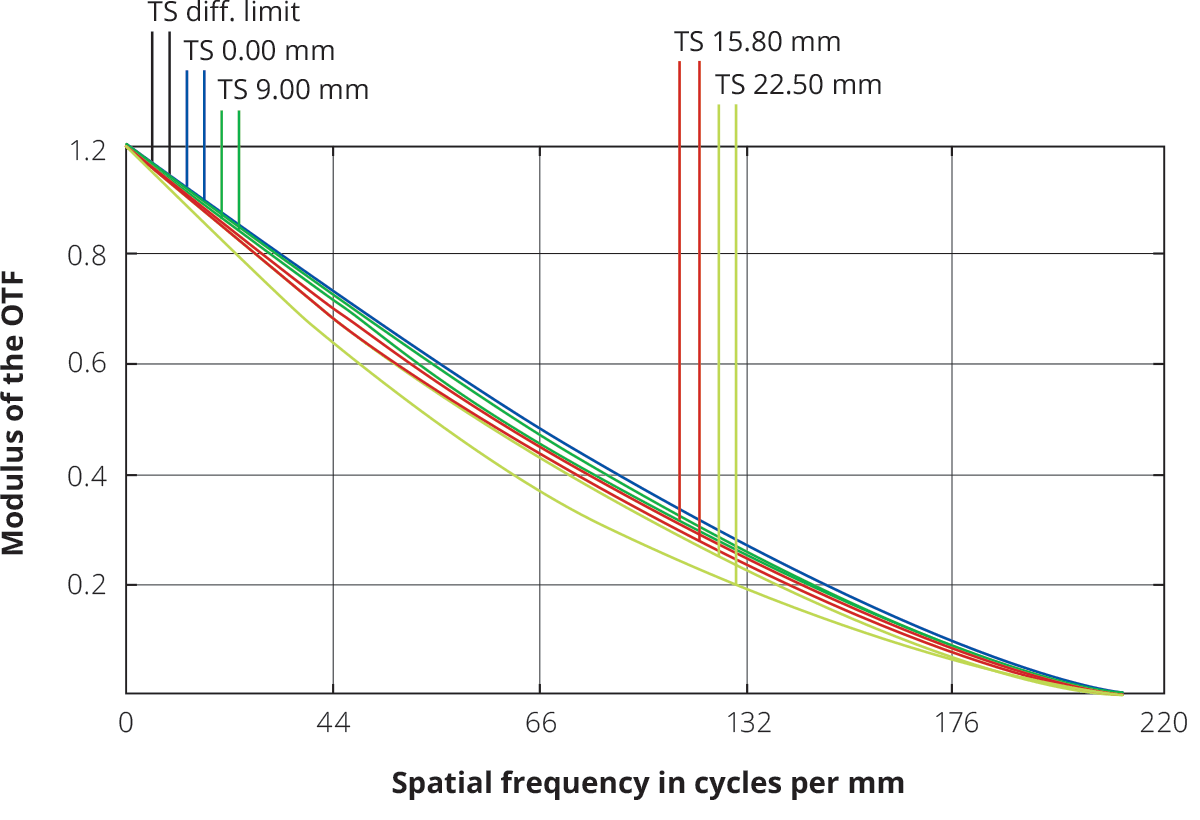# Lens resolving power: transfer function

The image quality of an optical system is usually expressed by its transfer function (TF). TF describes the ability of a lens to resolve features, correlating the spatial information in object space (usually expressed in line pair per millimeter) to the contrast achieved in the image.What's the difference between MTF (Modulation Transfer Function) and CTF (Contrast Transfer Function)? CTF expresses the lens contrast response when a “square pattern” (chessboard style) is imaged; this parameter is the most useful in order to assess edge sharpness for measurement applications. On the other hand, MTF is the contrast response achieved when imaging a sinusoidal pattern in which the grey levels range from 0 and 255; this value is more difficult to convert into any useful parameter for machine vision applications. The resolution of a lens is typically expressed by its MTF (modulation transfer function), which shows the response of the lens when a sinusoidal pattern is imaged.

However, the CTF (Contrast Transfer Function) is a more interesting parameter, because it describes the lens contrast when imaging a black and white stripe pattern, thus simulating how the lens would image the edge of an object. If t is the width of each stripe, the relative spatial frequency w will be

w = 1/(2t)

For example, a black and white stripe pattern with 5 µm wide stripes has a spatial frequency of 100 lp/mm. The “cut-off frequency” is defined as the value w for which CTF is zero, and it can be estimated as

w_("cutoff") = 1/[WF//# * λ(mm)]

For example, an Opto Engineering® TC23036 lens (WF/#h F/8) operating in green light (λ = 0.000587 mm) has a cut-off spatial frequency of about

w_("cutoff") = 1/([ 8 * 0.000587 mm ]) = 213" "lp//mmNext →